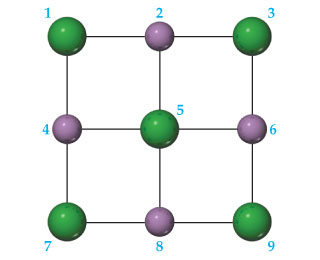# Problem: A portion of a two-dimensional "slab" of NaCl(s) is shown here in which the ions are numbered.If this pattern of ions was extended indefinitely in two dimensions, would the lattice energy be positive or negative?

###### FREE Expert Solution

We have to determine if the given “slab”, when extended indefinitely in two dimensions, would result into a positive or negative lattice energy.

Lattice energy is the energy released when gaseous ions are brought closer to form a crystalline solid.

81% (86 ratings)###### Problem Details
A portion of a two-dimensional "slab" of NaCl(s) is shown here in which the ions are numbered.If this pattern of ions was extended indefinitely in two dimensions, would the lattice energy be positive or negative?

Frequently Asked Questions

What scientific concept do you need to know in order to solve this problem?

Our tutors have indicated that to solve this problem you will need to apply the Lattice Energy concept. You can view video lessons to learn Lattice Energy. Or if you need more Lattice Energy practice, you can also practice Lattice Energy practice problems.